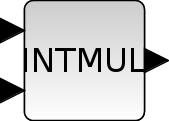Change language to:
Français - 日本語 - Português - Русский

See the recommended documentation of this function

Scilab Help >> Xcos > palettes > Integer palette > INTMUL

# INTMUL

Integer matrix multiplication

### Block Screenshot### Description

That block computes the matrix multiplication of two integer input matrices. The number of rows of the second matrix must be equal to the number of columns of the first matrix.

The output is a matrix where the number of rows is equal to the number of rows of the first input matrix and the number of columns is equal to the number of columns of the second input matrix.

On overflow, the result can take different forms:

1. A normal non saturated result. By example, if type is int8 and the result is 128, the block output value will be -128.

2. A saturated result. For the previous example the block output value will be 127

3. An error message warning the user about the overflow.

The user can select one of these three forms by setting the Do on Overflow field to 0, 1 or 2.

### Data types

The block supports the following types :

• Input : matrices, vectors or scalars. Scilab's integer data types (Data Type parameter).

• Output : same type that the inputs.

### Dialog box• Data Type (3:int32, 4:int16, 5:int8, ...)

It indicates the type of the input/output data : between 3 and 8.

Properties : Type 'vec' of size 1.

• Do on Overflow (0:Nothing, 1:Saturate, 2:Error)

Between 0 and 2. When there are an overflowing, if parameter is equal to :

• 0 : the result is non saturated.

• 1 : the result is saturated.

• 2 : the simulation displays en error message.

Properties : Type 'vec' of size 1.

### Default properties

• always active: no

• direct-feedthrough: yes

• zero-crossing: no

• mode: no

• regular inputs:

- port 1 : size [-1,-2] / type 3

- port 2 : size [-2,-3] / type 3

• regular outputs:

- port 1 : size [-1,-3] / type 3

• number/sizes of activation inputs: 0

• number/sizes of activation outputs: 0

• continuous-time state: no

• discrete-time state: no

• object discrete-time state: no

• name of computational function: matmul_i32

### Example

The goal of this example is to code two decimals digits in an only byte. It makes exactly the inverse work of the example of the EXTRACTBITS block.### Interfacing function

• SCI/modules/scicos_blocks/macros/IntegerOp/INTMUL.sci

### Computational function

• SCI/modules/scicos_blocks/src/c/matmul_i32n.c

• SCI/modules/scicos_blocks/src/c/matmul_i16n.c

• SCI/modules/scicos_blocks/src/c/matmul_i8n.c

• SCI/modules/scicos_blocks/src/c/matmul_ui32n.c

• SCI/modules/scicos_blocks/src/c/matmul_ui16n.c

• SCI/modules/scicos_blocks/src/c/matmul_ui8n.c

• SCI/modules/scicos_blocks/src/c/matmul_i32s.c

• SCI/modules/scicos_blocks/src/c/matmul_i16s.c

• SCI/modules/scicos_blocks/src/c/matmul_i8s.c

• SCI/modules/scicos_blocks/src/c/matmul_ui32s.c

• SCI/modules/scicos_blocks/src/c/matmul_ui16s.c

• SCI/modules/scicos_blocks/src/c/matmul_ui8s.c

• SCI/modules/scicos_blocks/src/c/matmul_i32e.c

• SCI/modules/scicos_blocks/src/c/matmul_i16e.c

• SCI/modules/scicos_blocks/src/c/matmul_i8e.c

• SCI/modules/scicos_blocks/src/c/matmul_ui32e.c

• SCI/modules/scicos_blocks/src/c/matmul_ui16e.c

• SCI/modules/scicos_blocks/src/c/matmul_ui8e.c